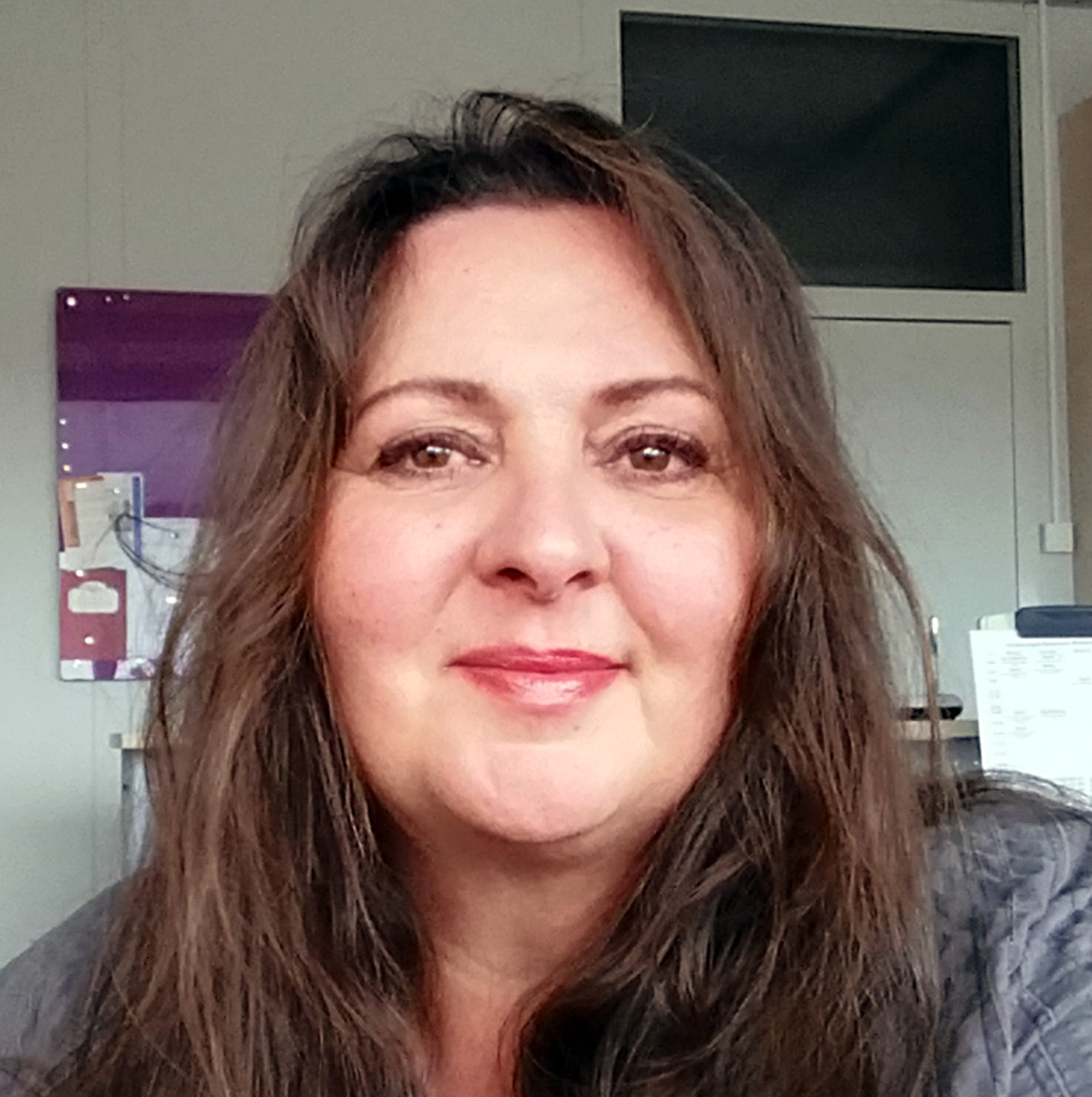# Research

Institute for Discrete Structures and Symbolic Computation

The IDSC at the University of Stuttgart conducts research in the fields of algebra and geometry incorporating methods of symbolic computation.

## Theoretical mathematics and symbolic computation

The aim of symbolic computation is to provide exact instead of numerical / approximative solutions. In this sense, symbolic computation is complementary to scientific computing. Symbolic computation allows, for instance, to carry out substantial experiments with abstract mathematical structures thus providing counterexamples to conjectures, specifications of working hypotheses or even hints to previously undiscovered relationships. Ultimately, symbolic computation helps to establish general theorems whose proof has been reduced to the consideration of finitely many cases. During the process new significant theoretical results as well as innovative algorithms often emerge which are helpful for further investigations. Finally, the term "discrete structures" underlines on one hand side the complementary character of symbolic computation compared to numerical-approximative methods. On the other, it encompasses the areas of mathematics in which algebraic and geometric methods are successfully applied.

### Our research focus

is set on the theory of finite and algebraic groups, algebraic geometry, and the development of constructive methods and efficient software. Among other things, we work on

• algebraic groups and finite groups of Lie type
• applications to theoretical biology and physics
• automorphism groups of algebraic and geometric objects
• Calabi-Yau and Hyperkähler varieties
• representation rings
• representation theory of finite groups
• unit groups of integer group rings
• lattices and crystallography
• Higgs bundles
• Kazhdan-Lusztig theory
• K3 and Enriques surfaces
• rational Cherednik algebras, symplectic mirror algebras and associated geometric structures
• mirror groups and Hecke algebras
• toric geometry

Further information on our research can be found on the personal pages of the institute members and on our research projects page.

### Contact: